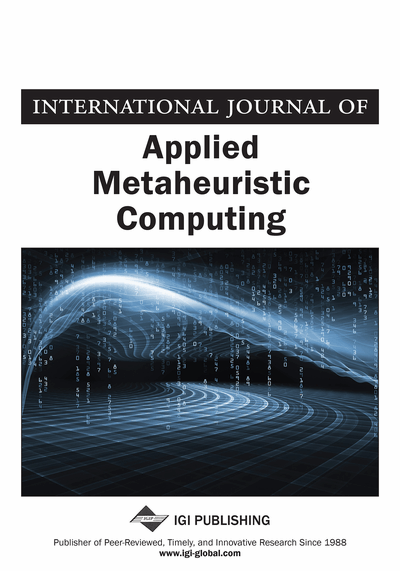# Environmental Adaption Method: A Heuristic Approach for Optimization

Anuj Chandila (IEC-CET, Greater Noida, India), Shailesh Tiwari (CSED, ABES Engineering College, Ghaziabad, India), K. K. Mishra (MNNIT Allahabad, India) and Akash Punhani (ABES Engineering College, Ghaziabad, India)
DOI: 10.4018/IJAMC.2019010107

## Abstract

This article describes how optimization is a process of finding out the best solutions among all available solutions for a problem. Many randomized algorithms have been designed to identify optimal solutions in optimization problems. Among these algorithms evolutionary programming, evolutionary strategy, genetic algorithm, particle swarm optimization and genetic programming are widely accepted for the optimization problems. Although a number of randomized algorithms are available in literature for solving optimization problems yet their design objectives are same. Each algorithm has been designed to meet certain goals like minimizing total number of fitness evaluations to capture nearly optimal solutions, to capture diverse optimal solutions in multimodal solutions when needed and also to avoid the local optimal solution in multi modal problems. This article discusses a novel optimization algorithm named as Environmental Adaption Method (EAM) foable 3r solving the optimization problems. EAM is designed to reduce the overall processing time for retrieving optimal solution of the problem, to improve the quality of solutions and particularly to avoid being trapped in local optima. The results of the proposed algorithm are compared with the latest version of existing algorithms such as particle swarm optimization (PSO-TVAC), and differential evolution (SADE) on benchmark functions and the proposed algorithm proves its effectiveness over the existing algorithms in all the taken cases.
Article Preview
Top

## 1. Introduction

Optimization is a process of finding out the best solutions among all available solutions for a problem. In a given domain, selection of best solution is done on the basis of objective function. An optimal solution of a given problem will have either the maximum or minimum value of objective function. Thus, an optimization problem is a search problem in which optimization algorithm is used to target optimal solutions in the space of all possible solutions, known as problem search space. This search space may be continuous or discrete. Each point in this search space represents one solution.

Depending on the complexity of optimization problem deterministic or randomized version of optimization algorithms are designed. If numbers of points in the search space are less then dynamic programming can be applied to retrieve exact optimal solution. This algorithm extracts the best solution by comparing the fitness values of all possible solutions. Problems with large number of solutions will require many comparisons and it will be computationally infeasible to compare all those points to retrieve exact optimal solutions. For these NP Hard problems where even searching a better solution is very typical task, so a local optimal solution or nearly optimal solution may be very valuable. Thus these problems can be solved by local search algorithms, gradient based algorithm or randomized algorithms. Local search algorithms and gradient based algorithms use mathematical approach to target local optimal solutions. Randomized algorithms search in random direction until they are able to find out nearly optimal solutions. Many randomized algorithms such as evolutionary programming, evolutionary strategy, genetic algorithm, particle swarm optimization and genetic programming are widely acceptable for solving optimization problems.

Although a number of randomized algorithms are available in literature for solving optimization problems yet their design objectives are same. Each algorithm has been designed to meet certain goals like minimizing total number of fitness evaluations to capture nearly optimal solutions, to capture diverse optimal solutions in multi modal solutions when needed and also to escape from local optimal solution in multi modal problems (Elbeltagi, Hegazy & Grierson, 2005).

Proposed study discusses these objectives in detail, focus on solutions implemented by existing algorithms and finally design a novel algorithm.

The design objectives of all algorithms can be explained as follows.

### 1.1. Convergence Rate

The prime objective of a newly designed algorithm is to minimize the total number of fitness evaluations for capturing optimal solutions. This can be done by improving the convergence rate of new algorithm. Convergence rate of an algorithm denotes how fast an algorithm is approaching to the optimal solution. To improve the convergence rate of new algorithm one should know on which parameters the convergence rate depends and how it can be accelerated. After a intensive review of existing randomized algorithms, we have noticed that the convergence rate of these algorithm depends on the natural phenomena used for mapping to search optimal solution. This can be explained as follows

To solve complex optimization problems, one has to design a randomized algorithm which is capable to search optimal solution. So within randomized algorithm there has to be some logical steps that can guide the search. As in most of the cases nature of objective function is not known to the user, one should choose a mapping that can automatically guide the search even in absence of any information. Mapping of Natural phenomena provide such a framework for guiding the search toward optimal solution when search direction is not clear. This is the reason why most of these randomized algorithms are inspired by nature. So it can be inferred that searching capability of any nature inspired algorithm lies within the mapping used to implement natural phenomena. Hence convergence rate of any algorithm can be improved by mapping a new natural phenomenon that can target optimal solution as soon as possible. Even though many nature inspired algorithms exist still there is a need of new optimization algorithm that can capture optimal solution as early as possible.

## Complete Article List

Search this Journal:
Reset
Open Access Articles: Forthcoming
Volume 11: 4 Issues (2020): 2 Released, 2 Forthcoming
Volume 10: 4 Issues (2019)
Volume 9: 4 Issues (2018)
Volume 8: 4 Issues (2017)
Volume 7: 4 Issues (2016)
Volume 6: 4 Issues (2015)
Volume 5: 4 Issues (2014)
Volume 4: 4 Issues (2013)
Volume 3: 4 Issues (2012)
Volume 2: 4 Issues (2011)
Volume 1: 4 Issues (2010)
View Complete Journal Contents Listing